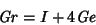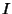## Griffiths Points

The'' Griffiths point is the fixed point in Griffiths' Theorem. Given four points on a Circle and a line through the center of the Circle, the four corresponding Griffiths points are Collinear (Tabov 1995).

The pointsare known as the first and second Griffiths points, whereis the Incenter and Ge is the Gergonne Point.

Oldknow, A. The Euler-Gergonne-Soddy Triangle of a Triangle.'' Amer. Math. Monthly 103, 319-329, 1996.
Tabov, J. Four Collinear Griffiths Points.'' Math. Mag. 68, 61-64, 1995.